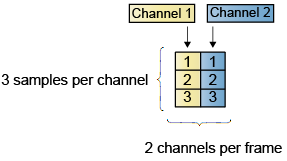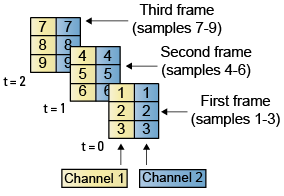## Import and Export Signals for Frame-Based Processing

In frame-based processing, blocks and System objects process data in frames. Each frame of data contains samples of consecutive times stacked together. Each channel is represented by a column of the input signal. For example, from a frame-based processing perspective, this 3-by-2 matrix has two channels, each containing three samples.When you configure a block to perform frame-based processing, the block interprets an M-by-1 vector as a single-channel signal containing M samples per frame. Similarly, the block interprets an M-by-N matrix as a multichannel signal with N independent channels and M samples per channel. For example, in frame-based processing, blocks interpret this sequence of 3-by-2 matrices as a two-channel signal with a frame size of 3.### Import Signals for Frame-Based Processing

The Signal From Workspace block creates a multichannel signal for frame-based processing when the Signal parameter is a matrix, and the Samples per frame parameter, M, is greater than `1`. Beginning with the first M rows of the matrix, the block releases M rows of the matrix (that is, one frame from each channel) to the output port every M*Ts seconds. Therefore, if the Signal parameter specifies a W-by-N workspace matrix, the Signal From Workspace block outputs a series of M-by-N matrices representing N channels. The workspace matrix must be oriented so that its columns represent the channels of the signal.

The figure below is a graphical illustration of this process for a 6-by-4 workspace matrix, `A`, and a frame size of 2.Note

Although independent channels are generally represented as columns, a single-channel signal can be represented in the workspace as either a column vector or row vector. The output from the Signal From Workspace block is a column vector in both cases.

In the following example, the Signal From Workspace block imports a three-channel signal comprising of two channels from the workspace matrix `dsp_examples_A` and one channel from the workspace column vector `dsp_examples_B`.

1. Open the Signal From Workspace Example 5 model by typing

`ex_importfbsigs`

at the MATLAB® command line.

```dsp_examples_A = [1:100;-1:-1:-100]'; % 100-by-2 matrix dsp_examples_B = 5*ones(100,1); % 100-by-1 column vector ```

The variable called dsp_examples_`A` represents a two-channel signal with 100 samples, and the variable called dsp_examples_`B` represents a one-channel signal with 100 samples.

Also, the following variables are defined in the MATLAB workspace:2. Double-click the Signal From Workspace block. Set the block parameters as follows, and then click :

• Signal parameter to ```[dsp_examples_A dsp_examples_B]```

• Sample time parameter to `1`

• Samples per frame parameter to `4`

• Form output after final data value parameter to ```Setting to zero```

Based on these parameters, the Signal From Workspace block outputs a signal with a frame size of 4 and a sample period of 1 second. The signal's frame period is 4 seconds. The Signal parameter uses the standard MATLAB syntax for horizontally concatenating matrices to append column vector `dsp_examples_B` to the right of matrix `dsp_examples_A`. After the block has output the signal, all subsequent outputs have a value of zero.

3. Run the model.

The figure below is a graphical representation of how your three-channel signal is imported into your model.You have now successfully imported a three-channel signal into your model using the Signal From Workspace block.

### Export Signals for Frame-Based Processing

The To Workspace (Simulink) and Triggered To Workspace blocks are the primary blocks for exporting signals of all dimensions from a Simulink® model to the MATLAB workspace.

A signal with N channels and frame size M is represented by a sequence of M-by-N matrices. When this signal is input to the To Workspace block, the block creates a P-by-N array in the MATLAB workspace containing the P most recent samples from each channel. The number of rows, P, is specified by the Limit data points to last parameter. The newest samples are added at the bottom of the matrix.

The following figure is a graphical illustration of this process for three consecutive frames of a signal with a frame size of 2 that is exported to matrix `A` in the MATLAB workspace.In the following example, you use a To Workspace block to export a three-channel signal with four samples per frame to the MATLAB workspace.

1. Open the Signal From Workspace Example 7 model by typing `ex_exportfbsigs` at the MATLAB command line.Also, the following variables are defined in the MATLAB workspace:

The variable called dsp_examples_`A` represents a two-channel signal with 100 samples, and the variable called dsp_examples_`B` represents a one-channel signal with 100 samples.

```dsp_examples_A = [1:100;-1:-1:-100]'; % 100-by-2 matrix dsp_examples_B = 5*ones(100,1); % 100-by-1 column vector ```
2. Double-click the Signal From Workspace block. Set the block parameters as follows, and then click :

• Signal = ```[dsp_examples_A dsp_examples_B]```

• Sample time = `1`

• Samples per frame = `4`

• Form output after final data value = `Setting to zero`

Based on these parameters, the Signal From Workspace block outputs a signal with a frame size of 4 and a sample period of 1 second. The signal's frame period is 4 seconds. The Signal parameter uses the standard MATLAB syntax for horizontally concatenating matrices to append column vector `dsp_examples_B` to the right of matrix `dsp_examples_A`. After the block has output the signal, all subsequent outputs have a value of zero.

3. Double-click the To Workspace block. Set the block parameters as follows, and then click :

• Variable name = `dsp_examples_yout`

• Limit data points to last = `inf`

• Decimation = `1`

• Frames = ```Concatenate frames (2-D array)```

Based on these parameters, the To Workspace block exports its input signal to a variable called `dsp_examples_yout` in the MATLAB workspace. The workspace variable can grow indefinitely large in order to capture all of the input data. The signal is not decimated before it is exported to the MATLAB workspace, and each input frame is vertically concatenated to the previous frame to produce a 2-D array output.

4. Run the model.

The following figure is a graphical representation of the model's behavior during simulation.5. At the MATLAB command line, type `dsp_examples_yout`.

The output is shown below:

```dsp_examples_yout = 1 -1 5 2 -2 5 3 -3 5 4 -4 5 5 -5 5 6 -6 5 7 -7 5 8 -8 5 9 -9 5 10 -10 5 11 -11 5 12 -12 5 ```

The frames of the signal are concatenated to form a two-dimensional array.

You have now successfully exported a signal to the MATLAB workspace using the To Workspace block.